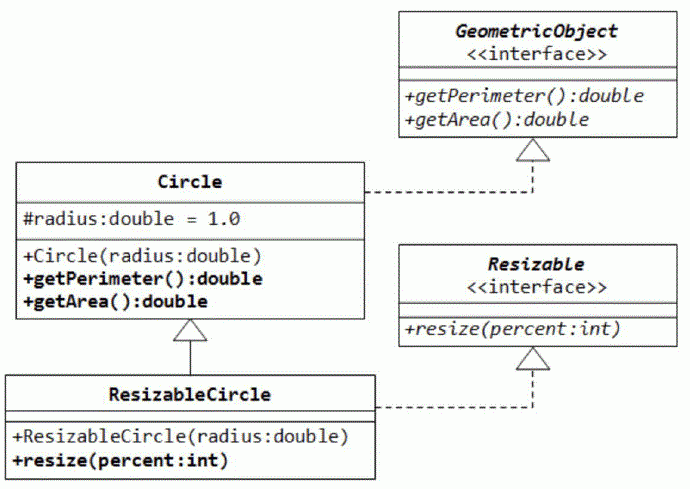favorite We need a little bit of your help to keep things running, click on this banner to learn more
Competitions

# Java Geometric Object

Write an application for the following UML diagram:• Write the interface called GeometricObject, which declares two methods: getParameter() and getArea();
• Write the implementation class Circle, with a protected variable radius, which implements the interface GeometricObject;
• The class ResizableCircle is defined as a subclass of the class Circle, which also implements an interface called Resizable. The interface Resizable declares a method resize(), which modifies the dimension (such as radius) by the given percentage. Write the interface Resizable and the class ResizableCircle;
• Write a test program to test the methods defined in ResizableCircle.

Process the next instructions:

• Area - print the area of the circle;
• Perimeter - print the circumference of the circle;
• Resize percent - resize the radius of the circle that modifies the radius by the given percentage;

Initially the radius of the circle equals to 1.

Resize by 50 percent means decrease the radius twice. Resize by 200 percent means increase the radius twice.

#### Input

Each line contains one of the instructions:

• Area
• Perimeter
• Resize percent

#### Output

Print the output for each instruction.

Time limit 1 second
Memory limit 128 MiB
Input example #1
Area
Perimeter
Resize 50
Area
Perimeter

Output example #1
3.141593
6.283185
0.785398
3.141593

Author Michael Medvediev